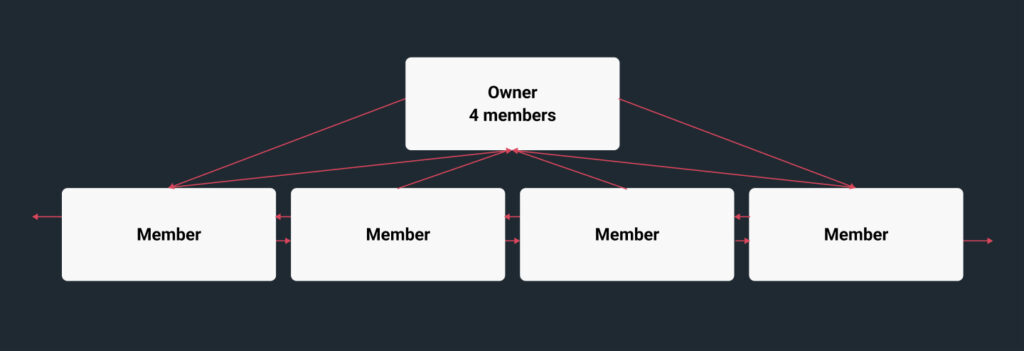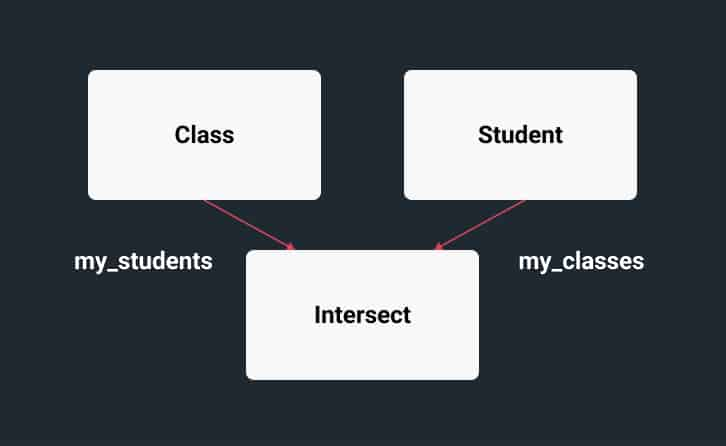# Advanced Data Modeling

### Relational Model

The most commonly understood and used data model today is the relational model where all data is defined in terms of tables and columns. RDM allows a database to be defined using SQL the predominant relation database language. Relationships in a pure relations model are defined by comparing column values in one table to common values in another. Indexing is a common method to optimize the comparisons. RDM supports both B+ tree, AVL tree, R Tree and Hash index implementations.

### Network Model

Beneath the relational model in an RDM database is a network model, where all data is defined in terms of record types and fields. Fields may be indexed and record types may have set relationships between them, which are defined as one-to-many, owner/member relationships. Set relationships occupy space in the records, stored in the data files. The owner record will contain pointers to member records. Member records will contain pointers to the owner record, plus the next and previous members. This allows for quick navigation among the members of a set and is conceptually very similar to a doubly linked list data structure. RDM uses the set construct to represent relational equi-joins.### Modeling Example

We will model a simple example for a school where we have students and classes. Students will take multiple classes and classes will be attended by multiple students. Therefore we have a many-to-many relationship between the student table and the class table. In the relational model a many-to-many relationship is modeled using an intersection record.The resulting database will have:

```				```
create database students;
create table class (
class_id char(5) primary key,
class_name char(29)
);
create table student (
name char(35) primary key
);
create table intersect (
begin_date integer,
end_date integer,
status char(9),